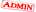# WAEC 2018 Chemistry practical Questions And ANSWERS Now Available– MAY/JUNE EXPO*WAEC 2018 CHEMISTRY PRACTICAL ANSWERS*

1)
Tabulate
Burette reading|Final burette reading
(cm^3)|Initial burette reading (cm^3)|
Volume of acid used(cm^3)|
Rough- 24.10,0.00,24.10
First- 23.80, 0.00, 23.80
Second- 23.75, 0.00, 23.70
Third- 23.75, 0.00, 23.75
Average volume of A used = 23.80 + 23.70 + 23.75cm^3/3
=23.75cm^3

==

1bi)
Con in mol/dm3=conc in g/dm^3/molar mass
con of B in g/dm^3 =4.80/0.25 =19.2g/dm^3
molar mass = Fe+2Cl = 56+2(35.5)=127g/mol
conc in mol/dm^3 =19.2/127 =0.15mol/dm^3
1bii)
using CAVA/CBVB = NA/NB
CA*11.80/0.15*20=1/5
CA=0.15*20*1/11.80*5
CA=0.05moldm^-3
1biii)
mole =con(mol/dm^3)x vol (dm^3)
=0.15*20/1000
=0.003moles

==

2a)
-Observation-
part of the C dissolves,colourless filtrate salt

-Inference-
C is a mixture of soluble salt

2bi)
-observation-
white precipitate formed

-Inference-
Cl is present

2bii)
-Observation-
precipitate dissolves

-Inference-
Cl confirmed

2biii)
-Observation-
colourless gas evolved with characteristic choking smell, turns litimus paper blue

-Inference-
Gas is NH3

2c)
-Observation-
Residue dissolves liberating a colourless gas which turns lime water milky

-Inference-
gas is CO2
CO^2-3 present

===

3a)A white precipitate of barium sulphate will be formed by the instant reaction between barium chloride and sulphuric acid.

BaCl2 + H2SO4 = BaSO4 + 2HCl

3b)Iron sulfide reacts with hydrochloric acid, releasing the malodorous (rotten egg smell) and very toxic gas,hydrogen sulphide. FeS + 2 HCl → FeCl2+ H2S

3c)When solid iron filings are added to dilute aqueous Hydrochloric acid, Iron (II)chloride or ferrous chloride is formed , with the liberation of Hydrogen gas.

Fe (s) + 2HCl (aq) ……..> FeCl2(aq) + H2 (g)

NOTE!!!
Always subscribe early!!!:-### Leave a ReplySubscribe
Notify of